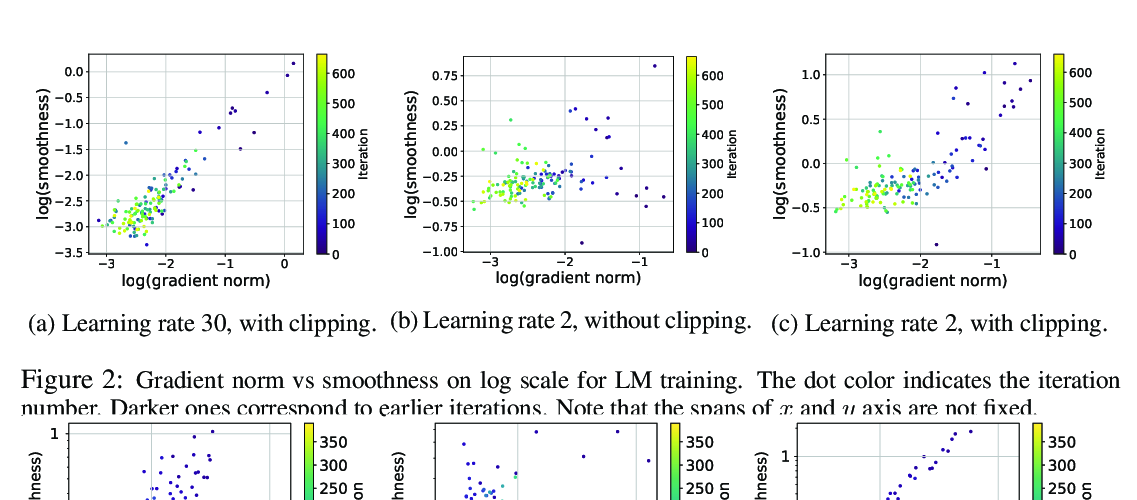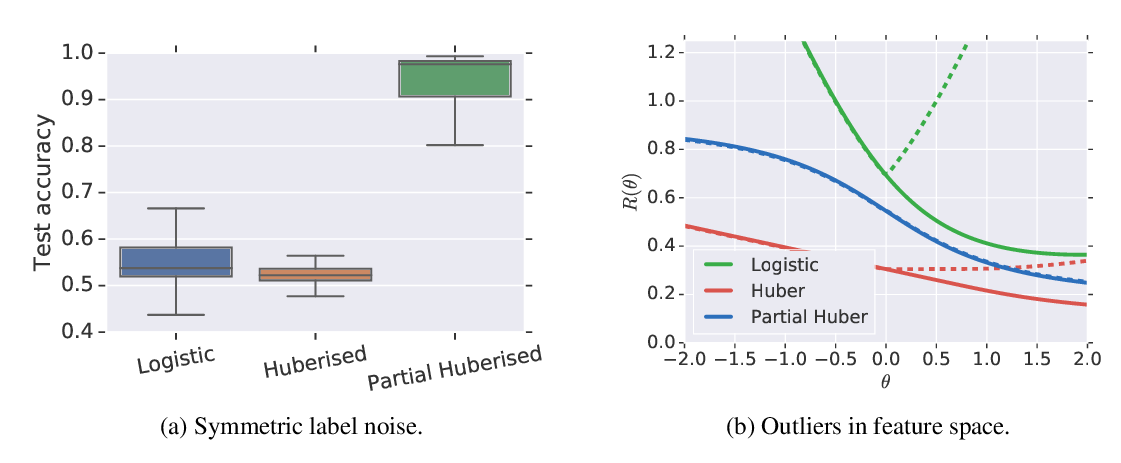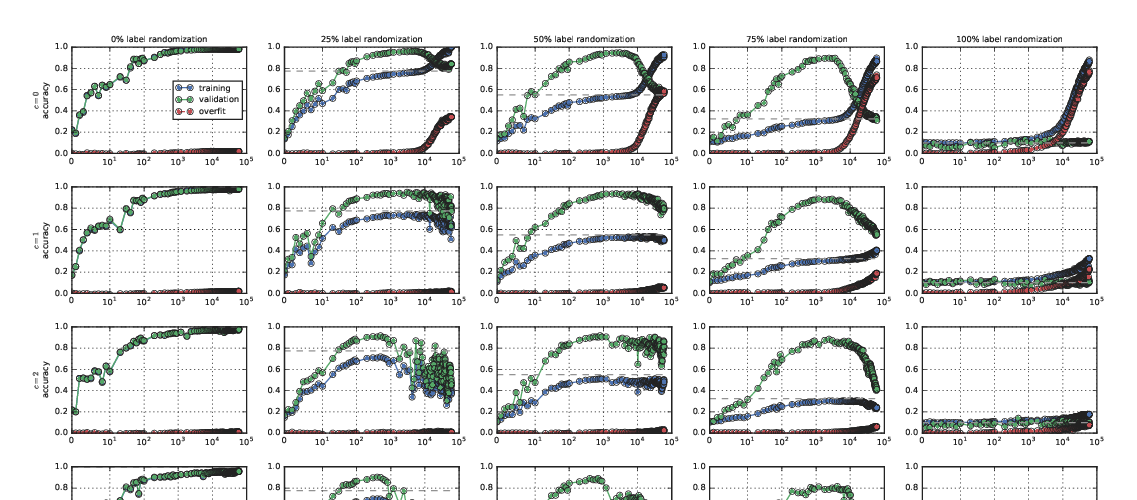## Gradient Descent Maximizes the Margin of Homogeneous Neural Networks

### Kaifeng Lyu, Jian Li

Wednesday: Theory

Abstract: In this paper, we study the implicit regularization of the gradient descent algorithm in homogeneous neural networks, including fully-connected and convolutional neural networks with ReLU or LeakyReLU activations. In particular, we study the gradient descent or gradient flow (i.e., gradient descent with infinitesimal step size) optimizing the logistic loss or cross-entropy loss of any homogeneous model (possibly non-smooth), and show that if the training loss decreases below a certain threshold, then we can define a smoothed version of the normalized margin which increases over time. We also formulate a natural constrained optimization problem related to margin maximization, and prove that both the normalized margin and its smoothed version converge to the objective value at a KKT point of the optimization problem. Our results generalize the previous results for logistic regression with one-layer or multi-layer linear networks, and provide more quantitative convergence results with weaker assumptions than previous results for homogeneous smooth neural networks. We conduct several experiments to justify our theoretical finding on MNIST and CIFAR-10 datasets. Finally, as margin is closely related to robustness, we discuss potential benefits of training longer for improving the robustness of the model.

## Similar Papers